Anúncio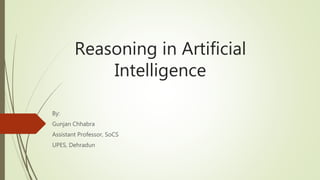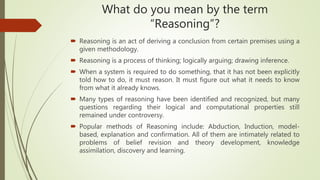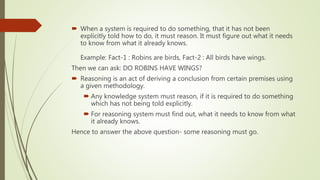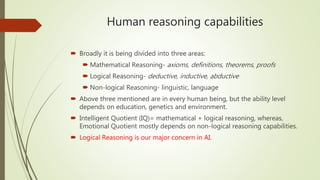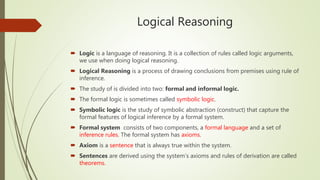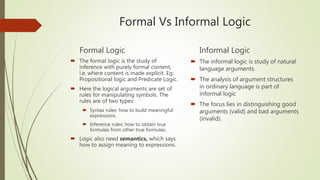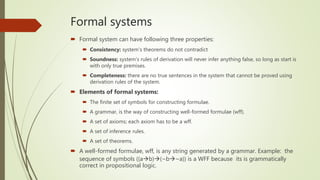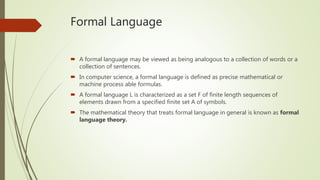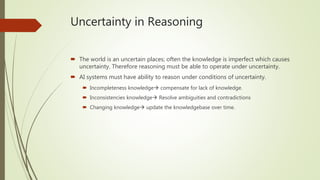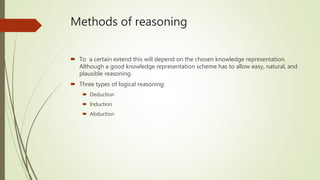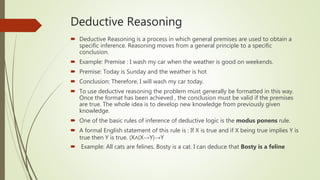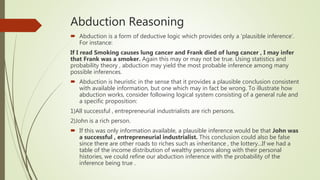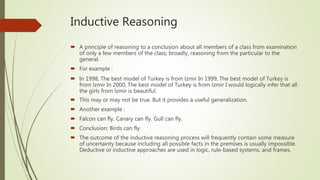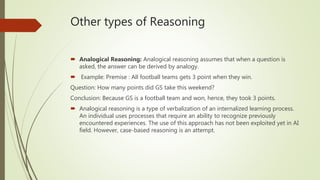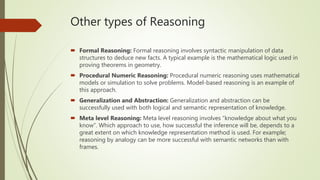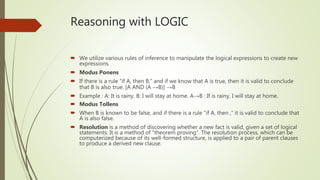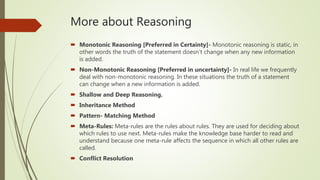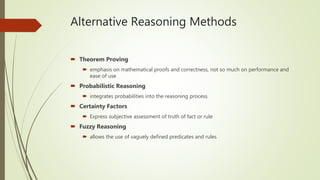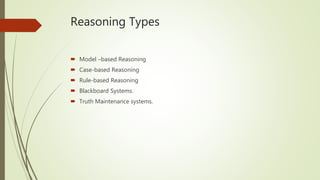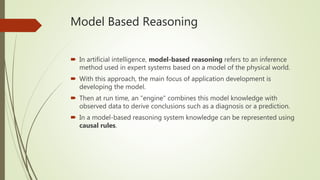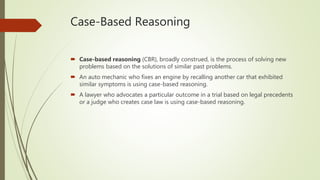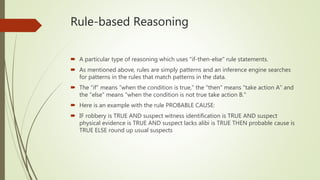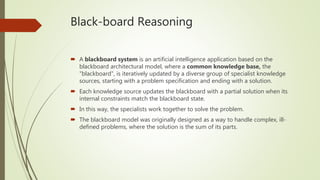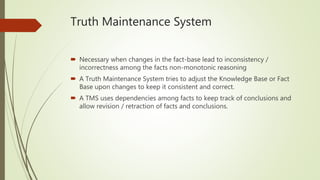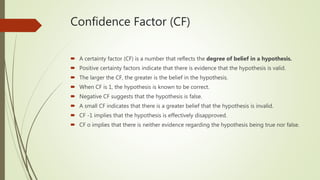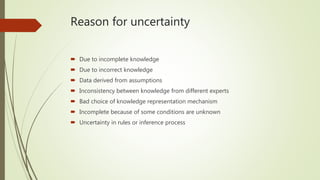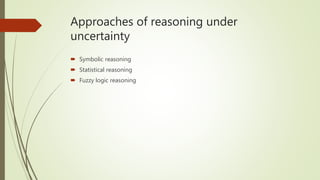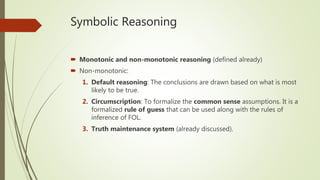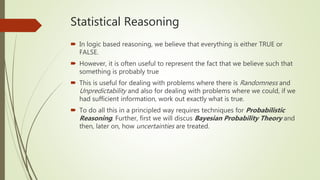1 de 29
Anúncio

### Reasoning in AI

1. Reasoning in Artificial Intelligence By: Gunjan Chhabra Assistant Professor, SoCS UPES, Dehradun
2. What do you mean by the term “Reasoning”?  Reasoning is an act of deriving a conclusion from certain premises using a given methodology.  Reasoning is a process of thinking; logically arguing; drawing inference.  When a system is required to do something, that it has not been explicitly told how to do, it must reason. It must figure out what it needs to know from what it already knows.  Many types of reasoning have been identified and recognized, but many questions regarding their logical and computational properties still remained under controversy.  Popular methods of Reasoning include: Abduction, Induction, model- based, explanation and confirmation. All of them are intimately related to problems of belief revision and theory development, knowledge assimilation, discovery and learning.
3.  When a system is required to do something, that it has not been explicitly told how to do, it must reason. It must figure out what it needs to know from what it already knows. Example: Fact-1 : Robins are birds, Fact-2 : All birds have wings. Then we can ask: DO ROBINS HAVE WINGS?  Reasoning is an act of deriving a conclusion from certain premises using a given methodology.  Any knowledge system must reason, if it is required to do something which has not being told explicitly.  For reasoning system must find out, what it needs to know from what it already knows. Hence to answer the above question- some reasoning must go.
4. Human reasoning capabilities  Broadly it is being divided into three areas:  Mathematical Reasoning- axioms, definitions, theorems, proofs  Logical Reasoning- deductive, inductive, abductive  Non-logical Reasoning- linguistic, language  Above three mentioned are in every human being, but the ability level depends on education, genetics and environment.  Intelligent Quotient (IQ)= mathematical + logical reasoning, whereas, Emotional Quotient mostly depends on non-logical reasoning capabilities.  Logical Reasoning is our major concern in AI.
5. Logical Reasoning  Logic is a language of reasoning. It is a collection of rules called logic arguments, we use when doing logical reasoning.  Logical Reasoning is a process of drawing conclusions from premises using rule of inference.  The study of is divided into two: formal and informal logic.  The formal logic is sometimes called symbolic logic.  Symbolic logic is the study of symbolic abstraction (construct) that capture the formal features of logical inference by a formal system.  Formal system consists of two components, a formal language and a set of inference rules. The formal system has axioms.  Axiom is a sentence that is always true within the system.  Sentences are derived using the system’s axioms and rules of derivation are called theorems.
6. Formal Vs Informal Logic Formal Logic  The formal logic is the study of inference with purely formal content, i.e. where content is made explicit. Eg: Propositional logic and Predicate Logic.  Here the logical arguments are set of rules for manipulating symbols. The rules are of two types:  Syntax rules: how to build meaningful expressions.  Inference rules: how to obtain true formulas from other true formulas.  Logic also need semantics, which says how to assign meaning to expressions. Informal Logic  The informal logic is study of natural language arguments.  The analysis of argument structures in ordinary language is part of informal logic  The focus lies in distinguishing good arguments (valid) and bad arguments (invalid).
7. Formal systems  Formal system can have following three properties:  Consistency: system’s theorems do not contradict  Soundness: system’s rules of derivation will never infer anything false, so long as start is with only true premises.  Completeness: there are no true sentences in the system that cannot be proved using derivation rules of the system.  Elements of formal systems:  The finite set of symbols for constructing formulae.  A grammar, is the way of constructing well-formed formulae (wff).  A set of axioms; each axiom has to be a wff.  A set of inference rules.  A set of theorems.  A well-formed formulae, wff, is any string generated by a grammar. Example: the sequence of symbols ((ab)(~b~a)) is a WFF because its is grammatically correct in propositional logic.
8. Formal Language  A formal language may be viewed as being analogous to a collection of words or a collection of sentences.  In computer science, a formal language is defined as precise mathematical or machine process able formulas.  A formal language L is characterized as a set F of finite length sequences of elements drawn from a specified finite set A of symbols.  The mathematical theory that treats formal language in general is known as formal language theory.
9. Uncertainty in Reasoning  The world is an uncertain places; often the knowledge is imperfect which causes uncertainty. Therefore reasoning must be able to operate under uncertainty.  AI systems must have ability to reason under conditions of uncertainty.  Incompleteness knowledge compensate for lack of knowledge.  Inconsistencies knowledge Resolve ambiguities and contradictions  Changing knowledge update the knowledgebase over time.
10. Methods of reasoning  To a certain extend this will depend on the chosen knowledge representation. Although a good knowledge representation scheme has to allow easy, natural, and plausible reasoning.  Three types of logical reasoning:  Deduction  Induction  Abduction
11. Deductive Reasoning  Deductive Reasoning is a process in which general premises are used to obtain a specific inference. Reasoning moves from a general principle to a specific conclusion.  Example: Premise : I wash my car when the weather is good on weekends.  Premise: Today is Sunday and the weather is hot  Conclusion: Therefore, I will wash my car today.  To use deductive reasoning the problem must generally be formatted in this way. Once the format has been achieved , the conclusion must be valid if the premises are true. The whole idea is to develop new knowledge from previously given knowledge.  One of the basic rules of inference of deductive logic is the modus ponens rule.  A formal English statement of this rule is : If X is true and if X being true implies Y is true then Y is true. (X∧(X→Y)→Y  Example: All cats are felines. Bosty is a cat. I can deduce that Bosty is a feline
12. Abduction Reasoning  Abduction is a form of deductive logic which provides only a ‘plausible inference’. For instance: If I read Smoking causes lung cancer and Frank died of lung cancer , I may infer that Frank was a smoker. Again this may or may not be true. Using statistics and probability theory , abduction may yield the most probable inference among many possible inferences.  Abduction is heuristic in the sense that it provides a plausible conclusion consistent with available information, but one which may in fact be wrong. To illustrate how abduction works, consider following logical system consisting of a general rule and a specific proposition: 1)All successful , entrepreneurial industrialists are rich persons. 2)John is a rich person.  If this was only information available, a plausible inference would be that John was a successful , entrepreneurial industrialist. This conclusion could also be false since there are other roads to riches such as inheritance , the lottery...If we had a table of the income distribution of wealthy persons along with their personal histories, we could refine our abduction inference with the probability of the inference being true .
13. Inductive Reasoning  A principle of reasoning to a conclusion about all members of a class from examination of only a few members of the class; broadly, reasoning from the particular to the general.  For example :  In 1998, The best model of Turkey is from İzmir In 1999, The best model of Turkey is from İzmir In 2000, The best model of Turkey is from İzmir I would logically infer that all the girls from İzmir is beautiful.  This may or may not be true. But it provides a useful generalization.  Another example :  Falcon can fly. Canary can fly. Gull can fly.  Conclusion: Birds can fly.  The outcome of the inductive reasoning process will frequently contain some measure of uncertainty because including all possible facts in the premises is usually impossible. Deductive or inductive approaches are used in logic, rule-based systems, and frames.
14. Other types of Reasoning  Analogical Reasoning: Analogical reasoning assumes that when a question is asked, the answer can be derived by analogy.  Example: Premise : All football teams gets 3 point when they win. Question: How many points did GS take this weekend? Conclusion: Because GS is a football team and won, hence, they took 3 points.  Analogical reasoning is a type of verbalization of an internalized learning process. An individual uses processes that require an ability to recognize previously encountered experiences. The use of this approach has not been exploited yet in AI field. However, case-based reasoning is an attempt.
15. Other types of Reasoning  Formal Reasoning: Formal reasoning involves syntactic manipulation of data structures to deduce new facts. A typical example is the mathematical logic used in proving theorems in geometry.  Procedural Numeric Reasoning: Procedural numeric reasoning uses mathematical models or simulation to solve problems. Model-based reasoning is an example of this approach.  Generalization and Abstraction: Generalization and abstraction can be successfully used with both logical and semantic representation of knowledge.  Meta level Reasoning: Meta level reasoning involves “knowledge about what you know”. Which approach to use, how successful the inference will be, depends to a great extent on which knowledge representation method is used. For example; reasoning by analogy can be more successful with semantic networks than with frames.
16. Reasoning with LOGIC  We utilize various rules of inference to manipulate the logical expressions to create new expressions.  Modus Ponens  If there is a rule “if A, then B,” and if we know that A is true, then it is valid to conclude that B is also true. [A AND (A →B)] →B  Example : A: It is rainy. B: I will stay at home. A→B : If is rainy, I will stay at home.  Modus Tollens  When B is known to be false, and if there is a rule "if A, then ,” it is valid to conclude that A is also false.  Resolution is a method of discovering whether a new fact is valid, given a set of logical statements. It is a method of “theorem proving”. The resolution process, which can be computerized because of its well-formed structure, is applied to a pair of parent clauses to produce a derived new clause.
17. More about Reasoning  Monotonic Reasoning [Preferred in Certainty]- Monotonic reasoning is static, in other words the truth of the statement doesn’t change when any new information is added.  Non-Monotonic Reasoning [Preferred in uncertainty]- In real life we frequently deal with non-monotonic reasoning. In these situations the truth of a statement can change when a new information is added.  Shallow and Deep Reasoning.  Inheritance Method  Pattern- Matching Method  Meta-Rules: Meta-rules are the rules about rules. They are used for deciding about which rules to use next. Meta-rules make the knowledge base harder to read and understand because one meta-rule affects the sequence in which all other rules are called.  Conflict Resolution
18. Alternative Reasoning Methods  Theorem Proving  emphasis on mathematical proofs and correctness, not so much on performance and ease of use  Probabilistic Reasoning  integrates probabilities into the reasoning process  Certainty Factors  Express subjective assessment of truth of fact or rule  Fuzzy Reasoning  allows the use of vaguely defined predicates and rules
19. Reasoning Types  Model –based Reasoning  Case-based Reasoning  Rule-based Reasoning  Blackboard Systems.  Truth Maintenance systems.
20. Model Based Reasoning  In artificial intelligence, model-based reasoning refers to an inference method used in expert systems based on a model of the physical world.  With this approach, the main focus of application development is developing the model.  Then at run time, an "engine" combines this model knowledge with observed data to derive conclusions such as a diagnosis or a prediction.  In a model-based reasoning system knowledge can be represented using causal rules.
21. Case-Based Reasoning  Case-based reasoning (CBR), broadly construed, is the process of solving new problems based on the solutions of similar past problems.  An auto mechanic who fixes an engine by recalling another car that exhibited similar symptoms is using case-based reasoning.  A lawyer who advocates a particular outcome in a trial based on legal precedents or a judge who creates case law is using case-based reasoning.
22. Rule-based Reasoning  A particular type of reasoning which uses "if-then-else" rule statements.  As mentioned above, rules are simply patterns and an inference engine searches for patterns in the rules that match patterns in the data.  The "if" means "when the condition is true," the "then" means "take action A" and the "else" means "when the condition is not true take action B."  Here is an example with the rule PROBABLE CAUSE:  IF robbery is TRUE AND suspect witness identification is TRUE AND suspect physical evidence is TRUE AND suspect lacks alibi is TRUE THEN probable cause is TRUE ELSE round up usual suspects
23. Black-board Reasoning  A blackboard system is an artificial intelligence application based on the blackboard architectural model, where a common knowledge base, the "blackboard", is iteratively updated by a diverse group of specialist knowledge sources, starting with a problem specification and ending with a solution.  Each knowledge source updates the blackboard with a partial solution when its internal constraints match the blackboard state.  In this way, the specialists work together to solve the problem.  The blackboard model was originally designed as a way to handle complex, ill- defined problems, where the solution is the sum of its parts.
24. Truth Maintenance System  Necessary when changes in the fact-base lead to inconsistency / incorrectness among the facts non-monotonic reasoning  A Truth Maintenance System tries to adjust the Knowledge Base or Fact Base upon changes to keep it consistent and correct.  A TMS uses dependencies among facts to keep track of conclusions and allow revision / retraction of facts and conclusions.
25. Confidence Factor (CF)  A certainty factor (CF) is a number that reflects the degree of belief in a hypothesis.  Positive certainty factors indicate that there is evidence that the hypothesis is valid.  The larger the CF, the greater is the belief in the hypothesis.  When CF is 1, the hypothesis is known to be correct.  Negative CF suggests that the hypothesis is false.  A small CF indicates that there is a greater belief that the hypothesis is invalid.  CF -1 implies that the hypothesis is effectively disapproved.  CF o implies that there is neither evidence regarding the hypothesis being true nor false.
26. Reason for uncertainty  Due to incomplete knowledge  Due to incorrect knowledge  Data derived from assumptions  Inconsistency between knowledge from different experts  Bad choice of knowledge representation mechanism  Incomplete because of some conditions are unknown  Uncertainty in rules or inference process
27. Approaches of reasoning under uncertainty  Symbolic reasoning  Statistical reasoning  Fuzzy logic reasoning
28. Symbolic Reasoning  Monotonic and non-monotonic reasoning (defined already)  Non-monotonic: 1. Default reasoning: The conclusions are drawn based on what is most likely to be true. 2. Circumscription: To formalize the common sense assumptions. It is a formalized rule of guess that can be used along with the rules of inference of FOL. 3. Truth maintenance system (already discussed).
29. Statistical Reasoning  In logic based reasoning, we believe that everything is either TRUE or FALSE.  However, it is often useful to represent the fact that we believe such that something is probably true  This is useful for dealing with problems where there is Randomness and Unpredictability and also for dealing with problems where we could, if we had sufficient information, work out exactly what is true.  To do all this in a principled way requires techniques for Probabilistic Reasoning. Further, first we will discus Bayesian Probability Theory and then, later on, how uncertainties are treated.
Anúncio